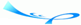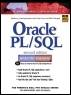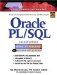# Chapter 2 PLSQL Concepts

 Team-FlyOracle® PL/SQL® Interactive Workbook, Second EditionBy Benjamin Rosenzweig, Elena Silvestrova Table of Contents Appendix D.  Answers to Test Your Thinking Sections

### Chapter 2 PL/SQL Concepts

 1) In order to calculate the area of a circle, the circle's radius must be squared and then multiplied by p. Write a program that calculates the area of a circle. The value for the radius should by provided with the help of a substitution variable. Use 3.14 for the value of p. Once the area of the circle is calculated, display it on the screen. A1: Answer: Your answer should look similar to the following: ` SET SERVEROUTPUT ON DECLARE v_radius NUMBER := &sv_radius; v_area NUMBER; BEGIN v_area := POWER(v_radius, 2) * 3.14; DBMS_OUTPUT.PUT_LINE ('The area of the circle is: '||v_area); END; `In this exercise, you declare two variables, v_radius and v_area, to store the values for the radius of the circle and its area, respectively. Next, you compute the value for the variable v_area with the help of the built-in function POWER and the value of the v_radius. Finally, you display the value of the v_area on the screen.Assume that number 5 has been entered for the value of the variable v_radius. Then the script produces the following output: ` Enter value for sv_radius: 5 old 2: v_radius NUMBER := &sv_radius; new 2: v_radius NUMBER := 5; The area of the circle is: 78.5 PLSQL procedure successfully completed. ` 2) Rewrite the script ch02_2b.sql, version 2.0. In the output produced by the script, extra spaces appear after the day of the week. The new script must remove the extra spaces after the day of the week. The current output: ` Today is Friday , 23:09 `The new output should have the format as shown: ` Today is Friday, 23:09 ` A2: Answer: Your answer should look similar to the following. All changes are shown in bold letters: ` SET SERVEROUTPUT ON DECLARE v_day VARCHAR2(20); BEGIN v_day := TO_CHAR(SYSDATE, 'fmDay, HH24:MI'); DBMS_OUTPUT.PUT_LINE ('Today is '|| v_day); END; `In this script, you modify the format in which you would like to display the date. Notice that the word 'Day' is now prefixed by the letters 'fm'. These letters guarantee that extra spaces will be removed from the name of the day. When run, this exercise produces the following output: ` Today is Tuesday, 18:54 PLSQL procedure successfully completed. `

 Team-FlyTopOracle PL/SQL Interactive Workbook (2nd Edition)
ISBN: 0130473200
EAN: 2147483647
Year: 2002
Pages: 146

Similar book on Amazon

flylib.com © 2008-2017.
If you may any questions please contact us: flylib@qtcs.net